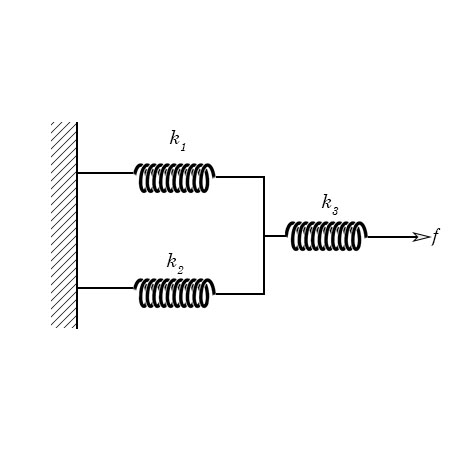# Determine the equivalent spring constant for the system shown below.

## Question:

Determine the equivalent spring constant for the system shown below.## Equivalent Spring Constant Computation:

Computation of equivalent spring constant, displacement and force for spring systems follow the Hooke's Law wherein it states that the force {eq}Fs {/eq} acted by the spring is equivalent to the product of the spring constant {eq}k {/eq} to the total displacement {eq}X {/eq} of the spring.

## Answer and Explanation: 1

Become a Study.com member to unlock this answer! Create your account

Given the spring system shown above, we are given three springs, k1, k2, and k3 arranged in a parallel and series connection.

Since springs k1 and k2...

See full answer below.

#### Learn more about this topic:Hooke's Law & the Spring Constant: Definition & Equation

from

Chapter 4 / Lesson 19
202K

After watching this video, you will be able to explain what Hooke's Law is and use the equation for Hooke's Law to solve problems. A short quiz will follow.